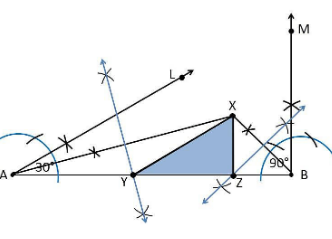# Construct a triangle XYZ in which ∠Y = 30°, ∠Z = 90° and XY + YZ + ZX = 11 cm.1. Draw a line segment AB which is equal to XY+YZ+ZX = 11 cm.

2. Make an angle ∠Y = 30° from point A and the angle be∠LAB

3. Make an angle ∠Z = 90° from the point B and the angle be ∠MAB

4. Bisect ∠LAB and ∠MAB at point X.

5 Considering the perpendicular bisector of the line XA and XB and the intersection point be Y and Z, respectively.

6. Join XY and XZ

7.Hence, XYZ is the required triangle